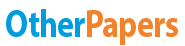# Salicylic Acid

Essay by   •  December 16, 2010  •  Term Paper  •  1,626 Words (7 Pages)  •  1,924 Views

## Essay Preview: Salicylic Acid

Report this essay
Page 1 of 7

Result

Table: Shows the absorbance value of different solution at pH1.

Solution Volume of Salicylic acid (ml) Volume of pH 1 buffer solution (ml) Absorbance

A 25 25 0.508

B 20 30 0.415

C 15 35 0.193

D 10 40 0.189

E 5 45 0.088

X (unknown) 0.534

Table: Shows the absorbance value of different solution at pH8.

Solution Volume of Salicylic acid (ml) Volume of pH 8 buffer solution(ml) Absorbance

A 25 25 0.370

B 20 30 0.298

C 15 35 0.214

D 10 40 0.138

E 5 45 0.066

X (unknown) 0.903

Calculation

Stock solution of Sodium Salicylate is 0.0005% (w/v) = 0.005g/100ml

Convert this into a molar concentration, using the following equation:

Molarity (M) = Fraction of 1L x (weight (g))/MW

= 1000/100 x 0.005/160.1

= 0.000312 M

Now, to calculate the concentration of the standards using: C1V1 = C2V2

Where,

C1 = concentration of the stock solution of sodium salicylate, 0.000312M as calculated above. This case C1 is kept the same throughout each calculation.

V1 = the volume of stock solution of salicylic acid, which would be different for each calculation being either 25, 20, 15, 10 or 5 cm3

C2 = the concentration of stock solution of salicylic acid

V2 = the total volume which is 50 cm3. This is kept constant throughout each calculation.

Solution A

C1=0.000312M V1=25 C2=? V2=50

C1V1=C2V2

0.000312 x 25 = C2 x 50

C2 = 1.56x10-4 M

Solution B

C1=0.000312M V2=20 C2=? V2=50

C1V1=C2V2

0.000312 x 20 =C2 x 50

C2 = 1.248x10-4 M

Solution C

C1= 0.000312M V2=15 C2=? V2=50

C1V1=C2V2

0.000312 x 15 =C2 x 50

C2 = 9.36x10-5 M

Solution D

C1= 0.000312M V2=10 C2=? V2=50

C1V1=C2V2

0.000312 x 10 =C2 x 50

C2 = 6.24x10-5 M

Solution E

C1= 0.000312M V2=5 C2=? V2=50

C1V1=C2V2

0.000312 x 5 =C2 x 50

C2 = 3.12x10-5 M

All the solutions A-E were then put through a spectrometer and the absorbance values were measured. Also there were two unknown solution, one for the pH1 and pH8 which were put through the spectrometer and the concentration of the two unknown solution had to be calculated.

By calculating the concentration of solution A-E a graph of absorbance against concentration is plotted from which the concentration of the unknown solution could be determined.

pH1 calculation

To make it easy, the concentration units is changed from M to μM.

Table: Shows the concentration and absorbance value at pH1.

Solution Concentration (μM) Absorbance

A 156 0.508

...

...